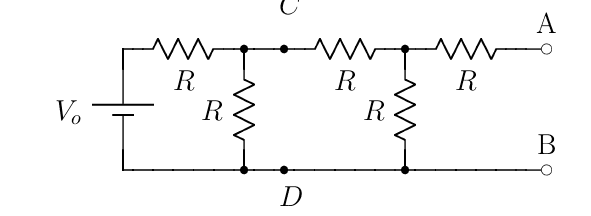# Open Circuit Voltage, Thevenin Equivalent

hbweb500

## Homework Statement

Find the open circuit voltage across A & B:V = IR

## The Attempt at a Solution

If I0 is the current leaving the voltage source and passing through the first resistor, I1 is the current through the left resistor in parallel, and I2 through the two other resistors, then:

$$V - I_0 R - I_1 R = 0$$

$$-2 I_2 R + I_1 R = 0$$

$$I_0 = I_1 + I_2$$

Solving gives a current $$I_2 = \frac{2 V}{5R}$$, therefore the potential difference across the A & B terminals is $$\Delta V = I_2 R = \frac{2 V}{5}$$

The book says this is [tex]\frac{V}{5}[tex].

Am I doing something wrong, or have I found an error in the book?

## Answers and Replies

willem2
You made an error while solving the equations. You can see this if you try to find all the currents: If I_2 = 2V/5R then I_1 is 4V/5R and I_0 wil be 6V/5R, but this means that the leftmost resistance has a potential difference of 1.2 V_0 across it, wich is impossible.

This problem is easier to solve if you compute the thevenin equivalent of the circuit to the left of C and D first. It will also be useful when you have to compute the short circuit current of the entire circuit.

hbweb500
Yep, that's the case. I must have plugged it into Mathematica wrong.